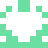### Real Vim ninjas count every keystroke - do you?

###### Pick a challenge, fire up Vim, and show us what you got.

```Your VimGolf key: please sign in

\$ gem install vimgolf
\$ vimgolf setup
\$ vimgolf put 9v0060a458ec000000000205
```

### Make list of numbers from 1 to 30 more compact, but more confusing

Change the naming logic of numbers to end up with less lines.

##### Start file
```one = 1
two = 2
three = 3
four = 4
five = 5
six = 6
seven = 7
eight = 8
nine = 9
ten = 10
eleven = 11
twelve = 12
thirteen = 13
fourteen = 14
fifteen = 15
sixteen = 16
seventeen = 17
eighteen = 18
nineteen = 19
twenty = 20
twenty_one = 21
twenty_two = 22
twenty_three = 23
twenty_four = 24
twenty_five = 25
twenty_six = 26
twenty_seven = 27
twenty_eight = 28
twenty_nine = 29
thirty = 30
```
##### End file
```one = 1
two = 2
three = 3
four = 4
five = 5
six = 6
seven = 7
eight = 8
nine = 9
ten = 10
eleven = [11, 12, 13]
tweleven = [14, 15, 16]
threleven = [17, 18, 19]
twenty = 20
twelve = [21, 22, 23]
twolve = [24, 25, 26]
threlve = [27, 28, 29]
thirty = 30
```

#### View Diff

```11,19c11,13
< eleven = 11
< twelve = 12
< thirteen = 13
< fourteen = 14
< fifteen = 15
< sixteen = 16
< seventeen = 17
< eighteen = 18
< nineteen = 19
---
> eleven = [11, 12, 13]
> tweleven = [14, 15, 16]
> threleven = [17, 18, 19]
21,29c15,17
< twenty_one = 21
< twenty_two = 22
< twenty_three = 23
< twenty_four = 24
< twenty_five = 25
< twenty_six = 26
< twenty_seven = 27
< twenty_eight = 28
< twenty_nine = 29
---
> twelve = [21, 22, 23]
> twolve = [24, 25, 26]
> threlve = [27, 28, 29]
```

### Solutions by @SidTweetsALess:

Unlock 1 remaining solutions by signing in and submitting your own entry

## 33 active golfers, 81 entries

##### Solutions by @SidTweetsALess:208
###### #31 - Siddharth Murali / @SidTweetsALess

07/03/2022 at 05:49PM